# Circle + square - math problems

#### Number of problems found: 90

• Square and circle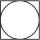Into square is inscribed circle with diameter 10 cm.What is difference between circumference square and circle?
• Cutting square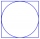From a square with a side of 30 cm, we cut the circle with the highest possible diameter. How many percents of the square content is this circle?
• Circumscribed circle to squareFind the length of a circle circumscribing a square of side 10 cm. Compare it to the perimeter of this square.
• Perimeter of the circleCalculate the perimeter of the circle in dm, whose radius equals the side of the square containing 0.49 dm2?
• CirclesArea of circle inscribed in a square is 14. What is the area of a circle circumscribed around a square?
• Squaring the Circle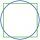Calculating side of the square with the same area as the circle of radius 18.
• Circle and squareAn ABCD square with a side length of 100 mm is given. Calculate the radius of the circle that passes through the vertices B, C and the center of the side AD.
• Circular ringSquare with area 16 centimeters square are inscribed circle k1 and described circle k2. Calculate the area of circular ring, which circles k1, k2 form.
• Square circles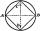Calculate the length of the described and inscribed circle to the square ABCD with a side of 5cm.
• MO circlesJuro built the ABCD square with a 12 cm side. In this square, he scattered a quarter circle that had a center at point B passing through point A and a semicircle l that had a center at the center of the BC side and passed point B. He would still build a c
• Ratio of squaresA circle is given in which a square is inscribed. The smaller square is inscribed in a circular arc formed by the side of the square and the arc of the circle. What is the ratio of the areas of the large and small squares?
• Larger perimeter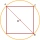There are a square and a circle that passes through two adjacent vertices of the square (end points of side a) and the center of the opposite side (c). Which of the plane shape has a larger perimeter?
• Circle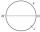On the circle k with diameter |MN| = 61 J lies point J. Line |MJ|=22. Calculate the length of a segment JN.
• InfinityIn a square with side 19 is inscribed circle, the circle is inscribed next square, again circle, and so on to infinity. Calculate the sum of the area of all these squares.
• Three shapes1/5 of a circle is shaded. The ratio of area if square to the sum of area of rectangle and that of the circle is 1:2. 60% of the square is shaded and 1/3 of the rectangle is shaded. What is the ratio of the area of circle to that of the rectangle?
• Circle chordWhat is the length x of the chord circle of diameter 115 m, if the distance from the center circle is 11 m?
• CenterCalculate the coordinates of the circle center: ?
• CircleWrite the equation of a circle that passes through the point [0,6] and touch the X-axis point [5,0]: ?
• Rectangle and circle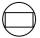The rectangle ABCD has side lengths a = 40 mm and b = 30 mm and is circumscribed by a circle k. Calculate approximately how many cm is circle long.
• Four circles1) Calculate the circle radius if its area is 400 cm square 2) Calculate the radius of the circle whose circumference is 400 cm. 3) Calculate circle circumference if its area is 400 cm square 4) Calculate the circle's area if perimeter 400 cm.

Do you have an interesting mathematical word problem that you can't solve it? Submit a math problem, and we can try to solve it.

We will send a solution to your e-mail address. Solved examples are also published here. Please enter the e-mail correctly and check whether you don't have a full mailbox.

Please do not submit problems from current active competitions such as Mathematical Olympiad, correspondence seminars etc...

Circle Problems. Square Problems.